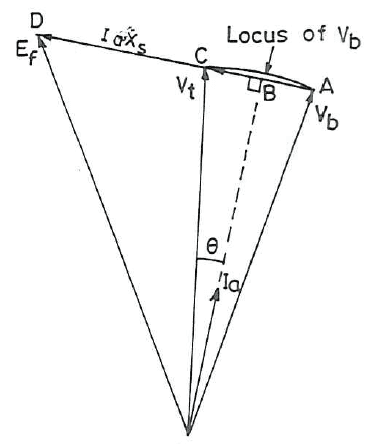# Synchronous generator phasor diagram

1. Problem Statement:
A 4-pole, star-connected, 50 Hz, 11kV, 40 MVA turbogenerator, with a synchronous reactance of 0.8 p.i., is connected to a power network. This power network can be represented by 11-kV infinite bus with a series reactance of j 0.5 Ω. A voltage regulator adjusts the field current such that alternator terminal voltage remains constant at 11 kV.

2. Relevant equation:
Based on the information above I can write the following equation:
$\overline{E}_ f= \underbrace{\overline{V}_{bus} + jX_{line}\overline{I}_a}_{V_t} + jX_{s}\overline{I}_a$

## The Attempt at a Solution

:[/B]
Based on the equation above I can draw the following phasor diagram. I just copied the one drawn in the book, due to my paint skills did not yield a pretty result.

The phasor diagrams draws $I_a$ lagging with respect to $V_t$, and therefore $\theta$ is the angle between them.

My question is: How do I know how to draw $I_a$? I know that $I_a$ should atleast be lagging $V_t$ at lagging power factor, but could it not also be drawn lagging with respect to $V_b$ and then $\theta$ is the angle between $V_b$ and $\theta$?

I guess $\theta$ is defined from $V_t$ is because $V_t$ is chosen as reference phasor. But I am still confused by how $I_a$ is placed.

Hope I made myself clear, and appriciate any help, best regards.